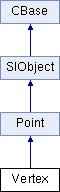﻿ C++: Vertex Class Reference

Vertex Class Reference

Vertex Class Reference

`#include <xsi_vertex.h>`

## Class Description

The Vertex object gives access to the geometry vertices of an X3DObject's Primitive. A Vertex is the object PolygonMesh specifics of the Point object.

You can access vertices from Edge::GetVertices, PolygonFace::GetVertices and PolygonMesh::GetVertices.

CVertexRefArray, Point, Edge, PolygonMesh, Geometry
Example:
```                using namespace XSI;
using namespace MATH;
Application app;
Model root = app.GetActiveSceneRoot();

X3DObject myCube;
root.AddGeometry( L"Cube", L"MeshSurface", L"", myCube );

PolygonMesh mesh(myCube.GetActivePrimitive().GetGeometry());
Vertex vertex0(mesh.GetVertices().GetItem(0));

app.LogMessage( L"Number of nodes for Vertex 0: " +
CValue(vertex0.GetNodes().GetCount()).GetAsText() );
```
Inheritance diagram for Vertex:List of all members.

## Public Member Functions

Vertex ()
~Vertex ()
Vertex (const CRef &in_ref)
Vertex (const Vertex &in_obj)
bool  IsA (siClassID in_ClassID) const
siClassID  GetClassID () const
Vertex operator= (const Vertex &in_obj)
Vertex operator= (const CRef &in_ref)
CPolygonNodeRefArray  GetNodes () const
CVertexRefArray  GetNeighborVertices (LONG in_lDistance=1) const
CEdgeRefArray  GetNeighborEdges (LONG in_lDistance=1) const
CPolygonFaceRefArray  GetNeighborPolygons (LONG in_lDistance=1) const
CVertexRefArray  GrowNeighborVertices (LONG in_lDistance=1) const
double  GetCrease () const
bool  GetIsBoundary () const

## Constructor & Destructor Documentation

 Vertex ( )

Default constructor.

 ~Vertex ( )

Default destructor.

 Vertex ( const CRef & in_ref )

Constructor.

Parameters:
 in_ref constant reference object.
 Vertex ( const Vertex & in_obj )

Copy constructor.

Parameters:
 in_obj constant class object.

## Member Function Documentation

 bool IsA ( siClassID in_ClassID ) const `[virtual]`

Returns true if a given class type is compatible with this API class.

Parameters:
 in_ClassID class type.
Returns:
true if the class is compatible, false otherwise.

Reimplemented from Point.

 siClassID GetClassID ( ) const `[virtual]`

Returns the type of the API class.

Returns:
The class type.

Reimplemented from Point.

 Vertex& operator= ( const Vertex & in_obj )

Creates an object from another object. The newly created object is set to empty if the input object is not compatible.

Parameters:
 in_obj constant class object.
Returns:
The new Vertex object.
 Vertex& operator= ( const CRef & in_ref )

Creates an object from a reference object. The newly created object is set to empty if the input reference object is not compatible.

Parameters:
 in_ref constant class object.
Returns:
The new Vertex object.

Reimplemented from Point.

 CPolygonNodeRefArray GetNodes ( ) const

Returns the PolygonNode objects adjacent to this Vertex.

Returns:
Array of PolygonNode objects.
 CVertexRefArray GetNeighborVertices ( LONG in_lDistance = `1` ) const

Returns an array of Vertex neighbors within a given distance. If the distance is 1 the Vertex are returned in counter-clockwise order.

Parameters:
 in_lDistance Value representing the degree of neighborhood
Returns:
Array of Vertex objects.
 CEdgeRefArray GetNeighborEdges ( LONG in_lDistance = `1` ) const

Returns an array of Edge neighbors within a given distance.If the distance is 1 all Edge objects for which one of the extremities is the current Vertex are returned in counter-clockwise order.

Parameters:
 in_lDistance Value representing the degree of neighborhood
Returns:
Array of Edge objects.
 CPolygonFaceRefArray GetNeighborPolygons ( LONG in_lDistance = `1` ) const

Returns an array of PolygonFace neighbors within a given distance.If the distance is 1 all PolygonFace for which one of the Vertex objects is the current Vertex are returned in counter-clockwise order.

Parameters:
 in_lDistance Value representing the degree of neighborhood
Returns:
Array of PolygonFace objects.
 CVertexRefArray GrowNeighborVertices ( LONG in_lDistance = `1` ) const

Returns an array of Vertex neighbors adjacent to this Vertex, within a given distance.

Parameters:
 in_lDistance Value representing the degree of neighborhood
Returns:
Array of Vertex objects.
 double GetCrease ( ) const

Returns the crease value associated with this vertex.

Returns:
The crease value between 0.0 and 10.0.
Since:
3.5.1
 bool GetIsBoundary ( ) const

Returns whether the control point is a boundary or not.

Returns:
true if the control point is a boundary, false if not.
Since:
4.2

The documentation for this class was generated from the following file: